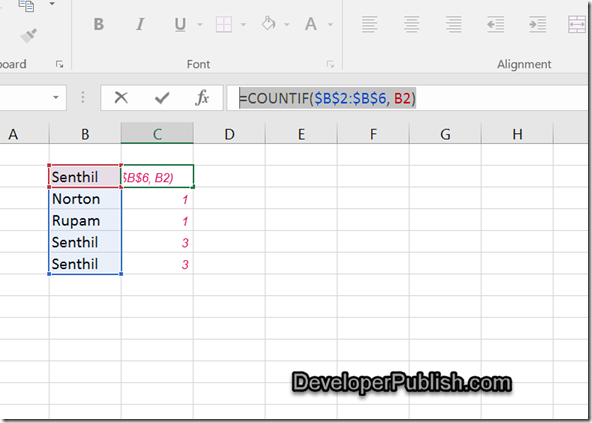# How to count the duplicate values in a column in Microsoft Excel 2016?

This blog post will explain in simple steps how you can count the duplicate values in a column in Microsoft Excel 2016.

Assume a scenario where you have a list of data in Column B in a worksheet that contains the name and you wish to count the frequency of duplicate values in it.

# How to count the duplicate values in a column in Microsoft Excel 2016?

You can use the COUNTIF function in Excel to perform this.

Assume that the cell range B2 to B6 contains the names and you would like to know how many times each of these values occur in the list. When the count of these values is greater than 2 , you can assume that they are duplicate values.Select the adjacent cell to B2 i.e C2 and enter the formula =COUNTIF(\$B\$2:\$B\$6, B2) and press enter key. Drag the Fill handle to the range of cells where you want to display the frequency for other cells.